# Performance Improvements by More Than 30% and a Data Race Fixed for CalculiX Code

Tuesday, February 2, 2021

CalculiX is an open source computational fluid dynamics code. It is performing finite element analysis to solve the computation problem iteratively until it reaches convergence. The application is written in C and Fortran and uses Pthread as its parallel programming model. For the analysis, our input data was a data sample that calculates the laminar flow of air through a bent pipe. The cross section of the pipe is square and the bend is 90 degrees, as illustrated in the figure below.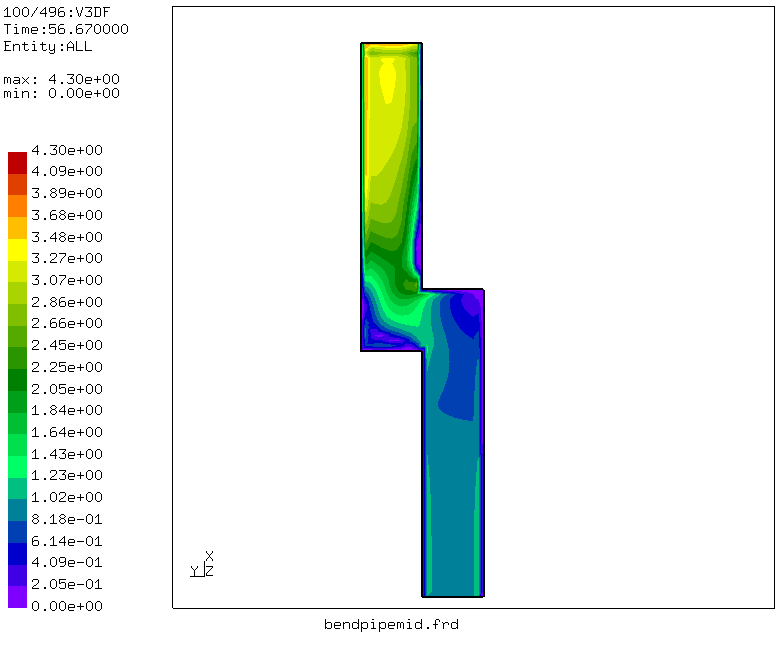Fig. 1  CalculiX input data showing the flow velocity within the pipe

During our initial examination of the application, we got nondeterministic results for executions with more than one thread. Using the correctness checkers Archer and Tsan, we performed correctness checking on the program and found a data race inside the CalculiX application. This data race was the source of nondeterminism in the calculation result output. After we fixed this problem, the application’s runtime and parallel efficiency showed much more reasonable values that can be seen from the figure below. In the original code that had the data race, when running with 8 threads, the CalculiX application can get more than 100% parallel efficiency.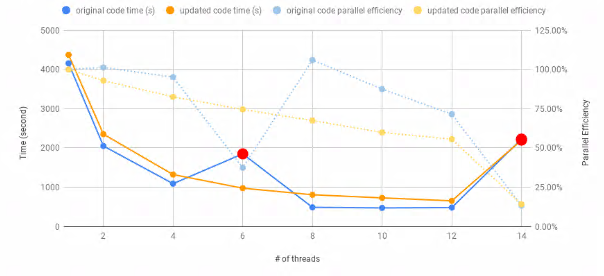Fig 2.  The original code shows much better execution time and parallel efficiency compared to the updated code because the data race made the application perform less number of iterations than it is supposed to do.

There are two major improvements that we made to the CalculiX application. One of the improvements is with its I/O write practice. CalculiX writes millions of small chunk log data continuously into the output file, and these writing activities account for almost 40% of the application's runtime. Using buffered I/O, we managed to improve the CalculiX execution runtime and speed up the application to more than 30% faster than the original execution time.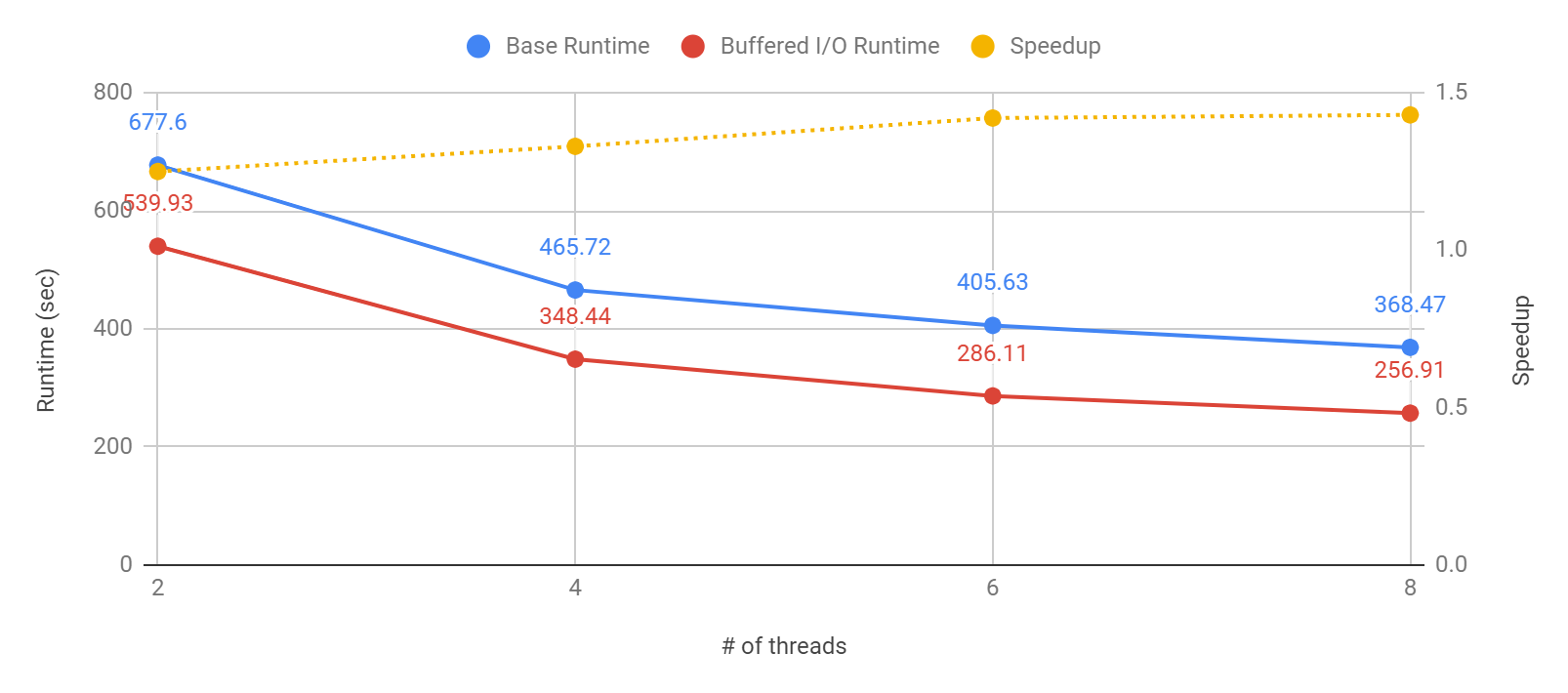Fig 3.  Runtime comparison before and after I/O optimization

Another major improvement was improving the load balance. From our initial evaluation, we have identified that CalculiX application’s main problem is load balance. In the figure below, we can see a Score-P trace comparison of the CalculiX-solver kernel pthread version (top) and the OpenMP version (bottom). Both versions show a similar pattern where one thread has more works compared to the other threads and causes the load imbalance.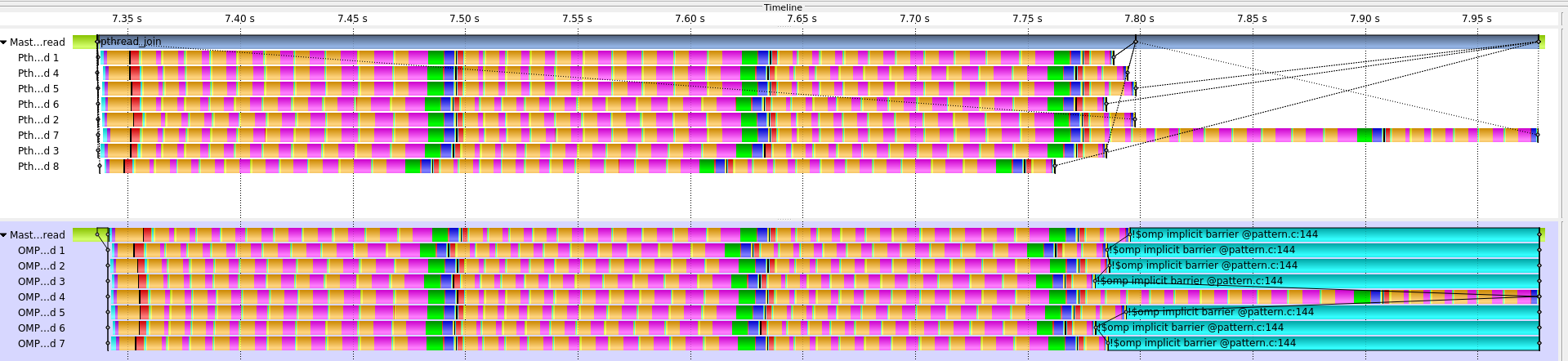Fig 4.  Score-P trace comparison of the CalculiX-solver kernel pthread version (top) and the OpenMP version (bottom)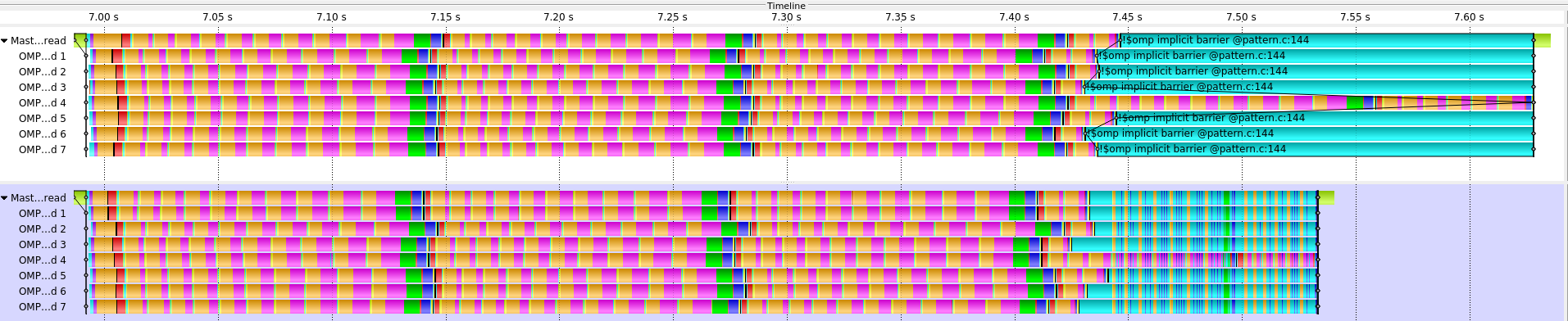Fig 5.  Score-P trace comparison of the CalculiX-solver pattern OpenMP version (top) and the implemented best practice using nested tasks (bottom)

The introduced solution shows an impact in the POP metrics where it increases the Global Efficiency by up to 17%. It is mainly driven by the Load Balance improvement (around 15% increase) even though we see decreases in Communication Efficiency (around 5% decrease) due to the nested task loop usage. We also get high numbers for Instruction Scalability due to the algorithm of the application. Since the large system matrix gets split into smaller independent subproblems (one for each thread) the overall computational work is changing depending on the numbers of threads. The smaller problems are a little easier to solve than the larger ones so they require fewer instructions.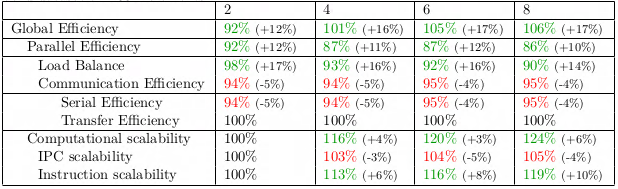Fig 6.  POP metrics numbers after implementing a nested task loop approach. The number inside the bracket is percentage improvement from the POP numbers of the original code

Combining the buffered I/O and nested task loop solution together, the overall performance improvement is even higher where we can get up to 24% improvement in the global efficiency as we can see from the POP metrics table below.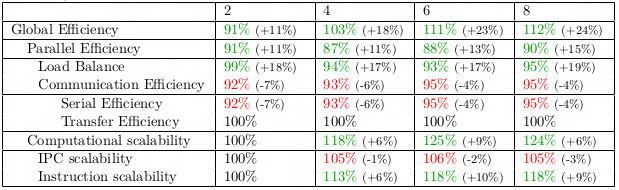Fig 7.  POP metrics numbers after implementing a nested task loop approach together with buffered I/O. The number inside the bracket is percentage improvement from the POP numbers of the original code

We also see a good result in the application’s runtime improvement from combining both improvement solutions we proposed in the POP Proof of Concept phase. Here we get up to 36% execution time improvement compared to the base result of the original code.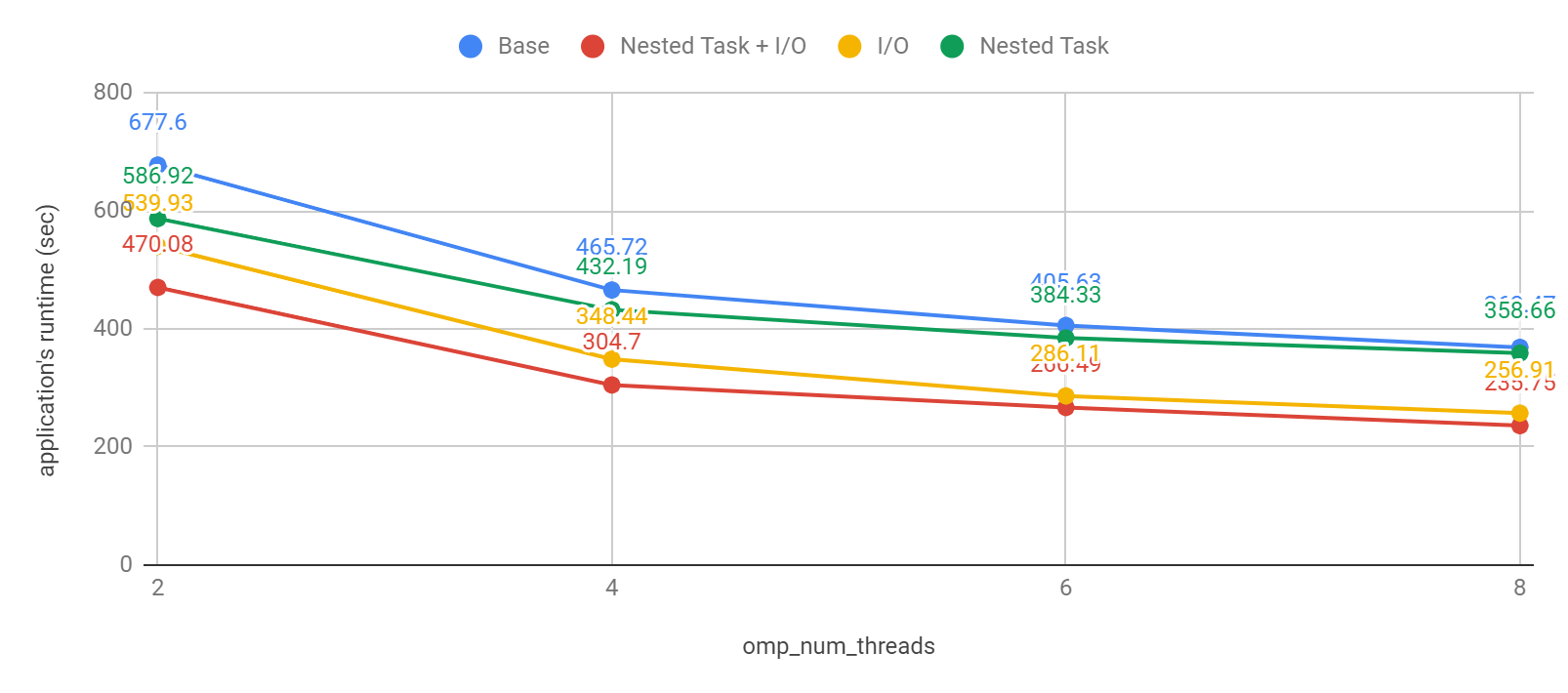Fig 8.  Performance comparsion of all program versions

Encouraged by this success, we are going forward with another analysis for Calculix as requested by the customer. This time we are examining the Characteristical Based Split method inside CalculiX. This project is still ongoing.

POP offers a thorough analysis of the customer’s code as a service, from ensuring the code’s correctness to analyzing performance efficiency with the POP methodology. The POP methodology provides customers with an easy to understand performance model to guide the performance optimization strategy.